CBSE (Science) Class 11CBSE
Share

# HC Verma solutions for Class 11 Physics chapter 13 - Magnetic Field due to a Current

#### HC Verma Concepts of Physics - Vol. 2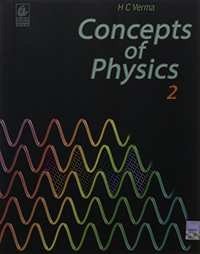## Chapter 13: Magnetic Field due to a Current

#### HC Verma solutions for Class 11 Physics Chapter 13 Exercise Short Answers [Page 248]

Short Answers | Q 1 | Page 248

An electric current flows in a wire from north to south. What will be the direction of the magnetic field due to this wire at a point (a) east of the wire, (b) west of the wire, (c) vertically above the wire and (d) vertically below the wire?

Short Answers | Q 2 | Page 248

The magnetic field due to a long straight wire has been derived in terms of µ, i and d. Express this in terms of ε0, c, i and d.

Short Answers | Q 3 | Page 248

You are facing a circular wire carrying an electric current. The current is clockwise as seen by you. Is the field at the centre coming towards you or going away from you?

Short Answers | Q 4 | Page 248

In Ampere's  $\oint \vec{B} \cdot d \vec{l} = \mu_0 i,$ the current outside the curve is not included on the right hand side. Does it mean  that the magnetic field B calculated by using Ampere's law, gives the contribution of only the currents crossing the area bounded by the curve?

Short Answers | Q 5 | Page 248

The magnetic field inside a tightly wound, long solenoid is B = µ0 ni. It suggests that the field does not depend on the total length of the solenoid, and hence if we add more loops at the ends of a solenoid the field should not increase. Explain qualitatively why the extra-added loops do not have a considerable effect on the field inside the solenoid.

Short Answers | Q 6 | Page 248

A long, straight wire carries a current. Is Ampere's law valid for a loop that does not enclose the wire, or that encloses the wire but is not circular?

Short Answers | Q 7 | Page 248

A straight wire carrying an electric current is placed along the axis of a uniformly charged ring. Will there be a magnetic force on the wire if the ring starts rotating about the wire? If yes, in which direction?

Short Answers | Q 8 | Page 248

Two wires carrying equal currents i each, are placed perpendicular to each other, just avoiding a contact. If one wire is held fixed and the other is free to move under magnetic forces, what kind of motion will result?

Short Answers | Q 9 | Page 248

Two proton beams going in the same direction repel each other whereas two wires carrying currents in the same direction attract each other. Explain.

Short Answers | Q 10 | Page 248

In order to have a current in a long wire, it should be connected to a battery or some such device. Can we obtain the magnetic due to a straight, long wire by using Ampere's law without mentioning this other part of the circuit?

Short Answers | Q 11 | Page 248

Quite often, connecting wires carrying currents in opposite directions are twisted together in using electrical appliances. Explain how it avoids unwanted magnetic fields.

Short Answers | Q 12 | Page 248

Two current-carrying wires may attract each other. In absence of other forces, the wires will moves towards each other increasing the kinetic energy. Does it contradict the fact that the magnetic force cannot do any work and hence cannot increase the kinetic energy?

#### HC Verma solutions for Class 11 Physics Chapter 13 Exercise MCQ [Pages 248 - 249]

MCQ | Q 1 | Page 248

A vertical wire carries a current in upward direction. An electron beam sent horizontally towards the wire will be deflected

•  towards right

• towards left

• upwards

• downwards.

MCQ | Q 2 | Page 248

A current-carrying, straight wire is kept along the axis of a circular loop carrying a current. This straight wire

• will exert an inward force on the circular loop

•  will exert an outward force on the circular loop

• will not exert any force on the circular loop

• will exert a force on the circular loop parallel to itself.

MCQ | Q 3 | Page 248

A proton beam is going from north to south and an electron beam is going from south to north. Neglecting the earth's magnetic field, the electron beam will be deflected

•  towards the proton beam

• away from the proton beam

• upwards

• downwards

MCQ | Q 4 | Page 248

A circular loop is kept in that vertical plane which contains the north-south direction. It carries a current that is towards north at the topmost point. Let A be a point on the axis of the circle to the east of it and B a point on this axis to the west of it. The magnetic field due to the loop

• is towards east at A and towards west at B

•  is towards west at A and towards east at B

•  is towards east at both A and B

• is towards west at both A and B

MCQ | Q 5 | Page 248

Consider the situation shown in figure. The straight wire is fixed but the loop can move under magnetic force. The loop will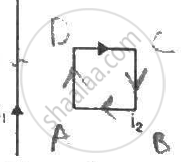• remain stationary

• move towards the wire

•  move away from the wire

MCQ | Q 6 | Page 248

A charged particle is moved along a magnetic field line. The magnetic force on the particle is

• along its velocity

•  opposite to its velocity

• perpendicular to its velocity

• zero

MCQ | Q 7 | Page 248

A moving charge produces

• electric field only

• magnetic field only

•  both of them

•  both of them

MCQ | Q 8 | Page 249

A particle is projected in a plane perpendicular to a uniform magnetic field. The area bounded by the path described by the particle is proportional to

• the velocity

• the momentum

• the kinetic energy

• none of these.

MCQ | Q 9 | Page 249

Two particles X and Y having equal charge, after being accelerated through the same potential difference enter a region of uniform magnetic field and describe circular paths of radii R1 and R2 respectively. The ratio of the mass of X to that of Y is

• (R1/R2)1/2

• R1/R2

•  (R1/R2)2

• R1R2.

MCQ | Q 10 | Page 249

Two parallel wires carry currents of 20 A and 40 A in opposite directions. Another wire carying a current anti parallel to 20 A is placed midway between the two wires. T he magnetic force on it will be

• towards 20 A

• towards 40 A

• zero

• perpendicular to the plane of the currents

MCQ | Q 11 | Page 249

Two parallel, long wires carry currents i1 and i2 with i1 > i2. When the currents are in the same direction, the magnetic field at a point midway between the wires is 10 µT. If the direction of i2 is reversed, the field becomes 30 µT. The ratio i1/i2 is

• 4

• 3

• 2

• 1

MCQ | Q 12 | Page 249

Consider a long, straight wire of cross-sectional area A carrying a current i. Let there be n free electrons per unit volume. An observer places himself on a trolley moving in the direction opposite to the current with a speed  $v = \frac{i}{\text{nAe}}$ and separation from the wire by a distance r. The magnetic field seen by the observer is very nearly

• $\frac{\mu_0 i}{2\pi r}$
• zero

• $\frac{\mu_0 i}{\pi r}$
• $\frac{2 \mu_0 i}{\pi r}$

#### HC Verma solutions for Class 11 Physics Chapter 13 Exercise MCQ [Page 249]

MCQ | Q 1 | Page 249

The magnetic field at the origin due to a current element  $i d \vec{l}$ placed at a position $\vec{r}$ is

(a)$\frac{\mu_0 i}{4\pi}\frac{d \vec{l} \times \vec{r}}{r^3}$

(b) $- \frac{\mu_0 i}{4\pi}\frac{\vec{r} \times d \vec{l}}{r^3}$

(c) $\frac{\mu_0 i}{4\pi}\frac{\vec{r} \times d \vec{l}}{r^3}$

(d) $- \frac{\mu_0 i}{4\pi}\frac{d \vec{l} \times \vec{r}}{r^3}$

MCQ | Q 2 | Page 249

Consider three quantities  $x = E/B, y = \sqrt{1/ \mu_0 \epsilon_0}$ and $z = \frac{l}{CR}$ . Here, l is the length of a wire, C is a capacitance and R is a resistance. All other symbols have standard meanings.

(a) xy have the same dimensions.
(b) yz have the same dimensions.
(c) zx have the same dimensions.
(d) None of the three pairs have the same dimensions.

MCQ | Q 3 | Page 249

A long, straight wire carries a current along the z-axis, One can find two points in the xy plane such that
(a) the magnetic fields are equal
(b) the directions of the magnetic fields are the same
(c) the magnitudes of the magnetic fields are equal
(d) the field at one point is opposite to that at the other point.

MCQ | Q 4 | Page 249

A long, straight wire of radius R carries a current distributed uniformly over its cross section. T he magnitude of the magnetic field is
(a) maximum at the axis of the wire
(b) minimum at the axis of the wire
(c) maximum at the surface of the wire
(d) minimum at the surface of the wire.

MCQ | Q 5 | Page 249

A hollow tube is carrying an electric current along its length distributed uniformly over its surface. The magnetic field
(a) increases linearly from the axis to the surface
(b) is constant inside the tube
(c) is zero at the axis
(d) is zero just outside the tube.

MCQ | Q 6 | Page 249

In a coaxial, straight cable, the central conductor and the outer conductor carry equal currents in opposite directions. The magnetic field is zero
(a) outside the cable
(b) inside the inner conductor
(c) inside the outer conductor
(d) in between the tow conductors.

MCQ | Q 7 | Page 249

A steady electric current is flowing through a cylindrical conductor.
(a) The electric field at the axis of the conductor is zero.
(b) The magnetic field at the axis of the conductor is zero.
(c) The electric field in the vicinity of the conductor is zero.
(d) The magnetic field in the vicinity of the conductor is zero.

#### HC Verma solutions for Class 11 Physics Chapter 13 [Pages 249 - 253]

Q 1 | Page 249

Using the formula $\vec{F} = q \vec{v} \times \vec{B} \text{ and } B = \frac{\mu_0 i}{2\pi r}$show that the SI units of the magnetic field B and the permeability constant µ0 may be written as N mA−1 and NA−2 respectively.

Q 2 | Page 249

A current of 10 A is established in a long wire along the positive z-axis. Find the magnetic field  $\vec{B}$  at the point (1 m, 0, 0).

Q 3 | Page 250

A copper wire of diameter 1.6 mm carries a current of 20 A. Find the maximum magnitude of the magnetic field  $\vec{B}$  due to this current.

Q 4 | Page 250

A transmission wire carries a current of 100 A. What would be the magnetic field B at a point on the road if the wire is 8 m above the road?

Q 5 | Page 250

A long, straight wire carrying a current of 1.0 A is placed horizontally in a uniform magnetic field B = 1.0 × 10−5 T pointing vertically upward figure. Find the magnitude of the resultant magnetic field at the points P and Q, both situated at a distance of 2.0 cm from the wire in the same horizontal plane.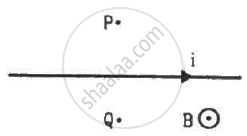Q 6 | Page 250

A long, straight wire of radius r carries a current i and is placed horizontally in a uniform magnetic field B pointing vertically upward. The current is uniformly distributed over its cross section. (a) At what points will the resultant magnetic field have maximum magnitude? What will be the maximum magnitude? (b) What will be the minimum  magnitude of the resultant magnetic field?

Q 7 | Page 250

A long, straight wire carrying a current of 30 A is placed in an external, uniform magnetic field of 4.0 × 10−4 T parallel to the current. Find the magnitude of the resultant magnetic field at a point 2.0 cm away from the wire.

Q 8 | Page 250

A long, vertical wire carrying a current of 10 A in the upward direction is placed in a region where a horizontal magnetic field of magnitude 2.0 × 10−3 T exists from south to north. Find the point where the resultant magnetic field is zero.

Q 9 | Page 250

Figure shows two parallel wires separated by a distance of 4.0 cm and carrying equal currents of 10 A along opposite directions. Find the magnitude of the magnetic field B at the points A1, A2, A3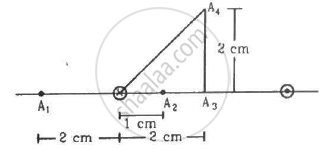Q 10 | Page 250

Two parallel wires carry equal currents of 10 A along the same direction and are separated by a distance of 2.0 cm. Find the magnetic field at a point which is 2.0 cm away from each of these wires.

Q 11 | Page 250

Two long, straight wires, each carrying a current of 5 A, are placed along the x- and y-axis respectively. The currents point along the positive directions of the axes. Find the magnetic fields at the points (a) (1 m, 1 m), (b) (−1 m, 1 m), (c) (−1 m, −1 m) and (d) (1 m, −1 m).

Q 12 | Page 250

Four long, straight wires, each carrying a current of 5.0 A, are placed in a plane as shown in figure. The points of intersection form a square of side 5.0 cm.
(a) Find the magnetic field at the centre P of the square.
(b) Q1, Q2, Q3, and Q4, are points situated on the diagonals of the square and at a distance from P that is equal to the diagonal of the square. Find the magnetic fields at these points.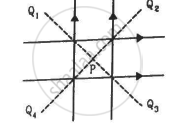Q 13 | Page 250

Figure shows a long wire bent at the middle to form a right angle. Show that the magnitudes of the magnetic fields at the point P, Q, R and S are equal and find this magnitude.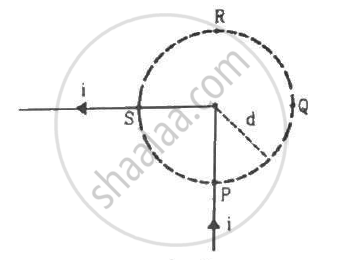Q 14 | Page 250

Consider a straight piece of length x of a wire carrying a current i. Let P be a point on the perpendicular bisector of the piece, situated at a distance d from its middle point. Show that for d >> x, the magnetic field at P varies as 1/d2 whereas for d << x, it varies as 1/d.

Q 15 | Page 250

Consider a 10-cm long piece of a wire which carries a current of 10 A. Find the magnitude of the magnetic field due to the piece at a point which makes an equilateral triangle with the ends of the piece.

Q 16 | Page 250

A long, straight wire carries a current i. Let B1 be the magnetic field at a point P at a distance d from the wire. Consider a section of length l of this wire such that the point P lies on a perpendicular bisector of the section B2 be the magnetic field at this point due to this second only. Find the value of d/l so that B2 differs from B1 by 1%.

Q 17 | Page 250

Figure shows a square loop ABCD with edge-length a. The resistance of the wire ABC is r and that of ADC is 2r. Find the magnetic field B at the centre of the loop assuming uniform wires.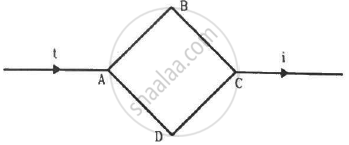Q 18 | Page 250

Figure shows a square loop of edge a made of a uniform wire. A current i enters the loop at the point A and leaves it at the point C. Find the magnetic field at the point P which is on the perpendicular bisector of AB at a distance a/4 from it.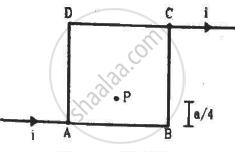Q 19 | Page 251

Consider the situation described in the previous problem. Suppose the current i enters the loop at the points A and leaves it at the point B. Find the magnetic field at the centre of the loop.

Q 20 | Page 251

The wire ABC shown in figure forms an equilateral triangle. Find the magnetic field B at the centre O of the triangle assuming the wire to be uniform.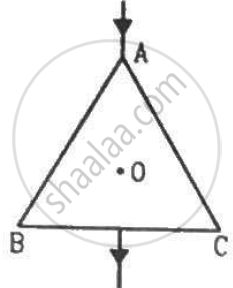Q 21 | Page 251

A wire of length l is bent in the form of an equilateral triangle and carries an electric current i. (a) Find the magnetic field B at the centre. (b) If the wire is bent in the form of a square, what would be the value of B at the centre?

Q 22 | Page 251

A long wire carrying a current i is bent to form a place along α . Find the magnetic field B at a point on the bisector of this angle situated at a distance x from the vertex.

Q 23 | Page 251

Find the magnetic field B at the centre of a rectangular loop of length l and width b, carrying a current i.

Q 24 | Page 251

A regular polygon of n sides is formed by bending a wire of total length 2πr which carries a current i. (a) Find the magnetic filed B at the centre of the polygon. (b) By letting n → ∞, deduce the expression for the magnetic field at the centre of a circular current.

Q 25 | Page 251

Each of the batteries shown in figure has an emf equal to 5 V. Show that the magnetic field B at the point P  is zero for any set of values of the resistances.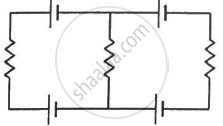Q 26 | Page 251

A straight, how wire carries a current of 20 A. Another wire carrying equal current is placed parallel to it. If the force acting on a length of 10 cm of the second wire is 2.0 × 10−5 N, what is the separation between them?

Q 27 | Page 251

Three coplanar parallel wires, each carrying a current of 10 A along the same direction, are placed with a separation 5.0 cm between the consecutive ones. Find the magnitude of the magnetic force per unit length acting on the wires.

Q 28 | Page 251

Two parallel wires separated by a distance of 10 cm carry currents of 10 A and 40 A along the same direction. Where should a third current by placed so that it experiences no magnetic force?

Q 29 | Page 251

Figure shows a part of an electric circuit. The wires AB, CD and EF are long and have identical resistance. The  separation between the neighbouring wires is 1.0 cm. The wires AE and BF have negligible resistance and the ammeter reads 30 A. Calculate the magnetic force per unit length of AB and CD.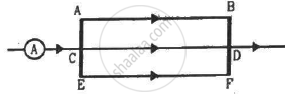Q 30 | Page 251

A long, straight wire is fixed horizontally and carries a current of 50.0 A. A second wire having linear mass density 1.0 × 10−4 kg m−1 is placed parallel to and directly above this wire at a separation of 5.0 mm. What current should this second wire carry such that the magnetic repulsion can balance its weight?

Q 31 | Page 251

A square loop PQRS carrying a current of 6.0 A is placed near a long wire carrying 10 A as shown in figure. (a) Show that the magnetic force acting on the part PQ is equal and opposite to the part RS. (b) Find the magnetic force on the square loop.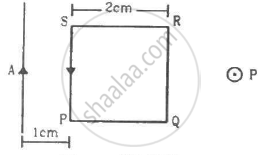Q 32 | Page 251

A circular loop of one turn carries a current of 5.00 A. If the magnetic field B at the centre is 0.200 mT, find the radius of the loop.

Q 33 | Page 251

A current-carrying circular coil of 100 turns and radius 5.0 cm produces a magnetic field of 6.0 × 10−5 T at its centre. Find the value of the current.

Q 34 | Page 251

An electron makes 3 × 105 revolutions per second in a circle of radius 0.5 angstrom. Find the magnetic field B at the centre of the circle.

Q 35 | Page 251

A conducting circular loop of radius a is connected to two long, straight wires. The straight wires carry a current i as shown in figure. Find the magnetic field B at the centre of the loop.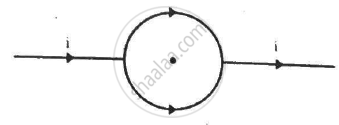Q 36 | Page 252

Two circular coils of radii 5.0 cm and 10 cm carry equal currents of 2.0 A. The coils have 50 and 100 turns respectively and are placed in such a way that their planes as well as the centres coincide. Find the magnitude of the magnetic field B at the common centre of the coils if the currents in the coils are (a) in the same sense (b) in the opposite sense.

Q 37 | Page 252

Two circular coils of radii 5.0 cm and 10 cm carry equal currents of 2.0 A. The coils have 50 and 100 turns respectively and are placed in such a way that their planes as well as the centres coincide. If the outer coil is rotated through 90° about a diameter, Find the  magnitude of the magnetic field B at the common centre of the coils if the currents in the coils are (a) in the same sense (b) in the opposite sense.

Q 38 | Page 252

A circular loop of radius 20 cm carries a current of 10 A. An electron crosses the plane of the loop with a speed of 2.0 × 106 m s−1. The direction of motion makes an angle of 30° with the axis of the circle and passes through its centre. Find the magnitude of the magnetic force on the electron at the instant it crosses the plane.

Q 39 | Page 252

A circular loop of radius R carries a current I. Another circular loop of radius r(<<R) carries a current i and is placed at the centre of the larger loop. The planes of the two circles are at right angle to each other. Find the torque acting on the smaller loop.

Q 40 | Page 252

A circular loop of radius r carrying a current i is held at the centre of another circular loop of radius R(>>r) carrying a current I. The plane of the smaller loop makes an angle of 30° with that of the larger loop. If the smaller loop is held fixed in this position by applying a single force at a point on its periphery, what would be the minimum magnitude of this force?

Q 41 | Page 252

Find the magnetic field B due to a semicircular wire of radius 10.0 cm carrying a current of 5.0 A at its centre of curvature.

Q 42 | Page 252

A piece of wire carrying a current of 6.00 A is bent in the form of a circular are of radius 10.0 cm, and it subtends an angle of 120° at the centre. Find the magnetic field B due to this piece of wire at the centre.

Q 43 | Page 252

A circular loop of radius r carries a current i. How should a long, straight wire carrying a current 4i be placed in the plane of the circle so that the magnetic field at the centre becomes zero?

Q 44 | Page 252

A circular coil of 200 turns has a radius of 10 cm and carries a current of 2.0 A. (a) Find the magnitude of the magnetic field $\vec{B}$ at the centre of the coil. (b) At what distance from the centre along the axis of the coil will the field B drop to half its value at the centre?

$(\sqrt{4} = 1 \cdot 5874 . . . )$
Q 45 | Page 252

A circular loop of radius 4.0 cm is placed in a horizontal plane and carries an electric current of 5.0 A in the clockwise direction as seen from above. Find the magnetic field (a) at a point 3.0 cm above the centre of the loop (b) at a point 3.0 cm below the centre of the loop.

Q 46 | Page 252

A charge of 3.14 × 10−6 C is distributed uniformly over a circular ring of radius 20.0 cm. The ring rotates about its axis with an angular velocity of 60.0 rad s−1. Find the ratio of the electric field to the magnetic field at a point on the axis at a distance of 5.00 cm from the centre.

Q 47 | Page 252

A thin but long, hollow, cylindrical tube of radius r carries i along its length. Find the magnitude  of the magnetic field at a distance r/2 from the surface (a) inside the tube (b) outside the tube.

Q 48 | Page 252

A long, cylindrical tube of inner and outer  radii a and b carries a current i distributed uniformly over its cross section. Find the magnitude of the magnitude filed at a point (a) just inside the tube (b) just outside the tube.

Q 49 | Page 252

A long, cylindrical wire of radius b carries a current i distributed uniformly over its cross section. Find the magnitude of the magnetic field at a point inside the wire at a distance a from the axis.

Q 50 | Page 252

A solid wire of radius 10 cm carries a current of 5.0 A distributed uniformly over its cross section. Find the magnetic field B at a point at a distance (a) 2 cm (b) 10 cm and (c) 20 cm away from the axis. Sketch a graph B versus x for 0 < x < 20 cm.

Q 51 | Page 252

Sometimes we show an idealised magnetic field which is uniform in a given region and falls to zero abruptly. One such field is represented in figure. Using Ampere's law over the path PQRS, show that such a field is not possible.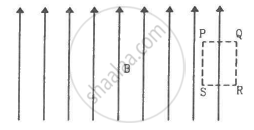Q 52 | Page 252

Two large metal sheets carry currents as shown in figure. The current through a strip of width dl is Kdl where K is a constant. Find the magnetic field at the points P, Q and R.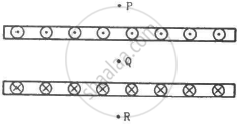Q 53 | Page 252

Consider the situation of the previous problem. A particle having charge q and mass mis projected from the point Q in a direction going into the plane of the diagram. It is found to describe a circle of radius r between the two plates. Find the speed of the charged particle.

Q 54 | Page 252

The magnetic field B inside a long solenoid, carrying a current of 5.00 A, is 3.14 × 10−2 T. Find the number of turns per unit length of the solenoid.

Q 55 | Page 252

A long solenoid is fabricated by closely winding a wire of radius 0.5 mm over a cylindrical nonmagnetic frame so that the successive turns nearly touch each other. What would be the magnetic field B at the centre of the solenoid if it carries a current of 5 A?

Q 56 | Page 252

A copper wire having resistance 0.01 ohm in each metre is used to wind a 400-turn solenoid of radius 1.0 cm and length 20 cm. Find the emf of a battery which when connected across the solenoid will cause a magnetic field of 1.0 × 10−2 T near the centre of the solenoid.

Q 57 | Page 253

A tightly-wound solenoid of radius a and length l has n turns per unit length. It carries an electric current i. Consider a length dx of the solenoid at a distance x from one end. This contains n dx turns and may be approximated as a circular current i n dx. (a) Write the magnetic field at the centre of the solenoid due to this circular current. Integrate this expression under proper limits to find the magnetic field at the centre of the solenoid. (b) verify that if l >> a, the field tends to B = µ0ni and if a >> l, the field tends to B =(mu_0nil)/(2a) . Interpret these results.

Q 58 | Page 253

A tightly-wound, long solenoid carries a current of 2.00 A. An electron is found to execute a uniform circular motion inside the solenoid with a frequency of 1.00 × 108 rev s−1. Find the number of turns per metre in the solenoid.

Q 59 | Page 253

A tightly-wound, long solenoid has n turns per unit length, a radius r and carries a current i. A particle having charge q and mass m is projected from a point on the axis in a direction perpendicular to the axis. What can be the maximum speed for which the particle does not strike the solenoid?

Q 60 | Page 253

A tightly-wound, long solenoid is kept with its axis parallel to a large metal sheet carrying a surface current. The surface current through a width dl of the sheet is Kdl and the number of turns per unit length of the solenoid is n. The magnetic field near the centre of the solenoid is found to be zero. (a) Find the current in the solenoid. (b) If the solenoid is rotated to make its axis perpendicular to the metal sheet, what would be the magnitude of the magnetic field near its centre?

Q 61 | Page 253

A capacitor of capacitance 100 µF is connected to a battery of 20 volts for a long time and then disconnected from it. It is now connected across a long solenoid having 4000 turns per metre. It is found that the potential difference across the capacitor drops to 90% of its maximum value in 2.0 seconds. Estimate the average magnetic field produced at the centre of the solenoid during this period.

## Chapter 13: Magnetic Field due to a Current

#### HC Verma Concepts of Physics - Vol. 2## HC Verma solutions for Class 11 Physics chapter 13 - Magnetic Field due to a Current

HC Verma solutions for Class 11 Physics chapter 13 (Magnetic Field due to a Current) include all questions with solution and detail explanation. This will clear students doubts about any question and improve application skills while preparing for board exams. The detailed, step-by-step solutions will help you understand the concepts better and clear your confusions, if any. Shaalaa.com has the CBSE Concepts of Physics - Vol. 2 solutions in a manner that help students grasp basic concepts better and faster.

Further, we at Shaalaa.com provide such solutions so that students can prepare for written exams. HC Verma textbook solutions can be a core help for self-study and acts as a perfect self-help guidance for students.

Concepts covered in Class 11 Physics chapter 13 Magnetic Field due to a Current are The Magnetic Dipole Moment of a Revolving Electron, Circular Current Loop as a Magnetic Dipole, Torque on a Rectangular Current Loop in a Uniform Magnetic Field, Magnetic Field on the Axis of a Circular Current Loop, Motion in a Magnetic Field, Velocity Selector, Solenoid and the Toroid - the Toroid, Solenoid and the Toroid - the Solenoid, Magnetic Diapole, Moving Coil Galvanometer, Torque on a Current Loop in Magnetic Field, Force Between Two Parallel Currents, the Ampere, Force on a Current - Carrying Conductor in a Uniform Magnetic Field, Cyclotron, Force on a Moving Charge in Uniform Magnetic and Electric Fields, Straight and Toroidal Solenoids (Only Qualitative Treatment), Ampere’s Circuital Law, Magnetic Field Due to a Current Element, Biot-Savart Law, Oersted’s Experiment, Magnetic Force.

Using HC Verma Class 11 solutions Magnetic Field due to a Current exercise by students are an easy way to prepare for the exams, as they involve solutions arranged chapter-wise also page wise. The questions involved in HC Verma Solutions are important questions that can be asked in the final exam. Maximum students of CBSE Class 11 prefer HC Verma Textbook Solutions to score more in exam.

Get the free view of chapter 13 Magnetic Field due to a Current Class 11 extra questions for Physics and can use Shaalaa.com to keep it handy for your exam preparation

S